• torch.matmul()没有强制规定维度和大小，可以用利用广播机制进行不同维度的相乘操作 当进行操作的两个tensor都是3D时，两者等同。 torch.bmm() 官网：https://pytorch.org/docs/stable/torch.html#torch.bmm ...
torch.bmm()
torch.matmul()
torch.bmm()强制规定维度和大小相同
torch.matmul()没有强制规定维度和大小，可以用利用广播机制进行不同维度的相乘操作
当进行操作的两个tensor都是3D时，两者等同。
torch.bmm()
torch.bmm(input, mat2, out=None) → Tensor
torch.bmm()是tensor中的一个相乘操作，类似于矩阵中的A*B。
参数：
input，mat2：两个要进行相乘的tensor结构，两者必须是3D维度的，每个维度中的大小是相同的。
output：输出结果
并且相乘的两个矩阵，要满足一定的维度要求：input（p,m,n) * mat2(p,n,a) ->output(p,m,a)。这个要求，可以类比于矩阵相乘。前一个矩阵的列等于后面矩阵的行才可以相乘。
torch.matmul() torch.matmul(input, other, out=None) → Tensor
torch.matmul()也是一种类似于矩阵相乘操作的tensor联乘操作。但是它可以利用python 中的广播机制，处理一些维度不同的tensor结构进行相乘操作。这也是该函数与torch.bmm()区别所在。
参数：
input,other：两个要进行操作的tensor结构
output:结果
举例：
a = torch.randn(2,3,2)
a = tensor([[[ 0.4198, -1.6376],          [-1.0197, -0.1295],          [-0.2412,  0.2189]],         [[-0.1045,  1.8026],          [-0.5264, -0.9585],          [ 2.4333, -0.3726]]])
b = torch.randn(2,1)
tensor([[-1.1622],         [-0.6326]])
torch.mm(a[0,:],b)
tensor([[0.5481],         [1.2670],         [0.1419]])
torch.mm(a[1,:],b)
tensor([[-1.0190],         [ 1.2181],         [-2.5922]])
c = torch.matmul(a,b)
c[0,:]=tensor([[0.5481],         [1.2670],         [0.1419]])
c[1,:]= tensor([[-1.0190],         [ 1.2181],         [-2.5922]])
展开全文pytorch一共有5种乘法
先定义下面的tensor（本文不展示print结果）：
import torch

tensorA_2x3 = torch.tensor(
[[1,2,3],
[3,2,1]]
)

tensorB_1x3 = torch.tensor(
[[1,2,3]]
)

tensorC_scalar = 5

tensorD_2x1 = torch.tensor(
[,
]
)

tensorE_3x1 = torch.tensor(
[,
,
]
)

tensorF_3x2 = torch.tensor(
[[1,2],
[3,4],
[5,6]]
)

tensorG_2x3x2 = torch.tensor(
[[[1,2],
[3,4],
[2,1]],
[[4,5],
[5,4],
[1,2]]]
)

tensorH_2x2x3 = torch.tensor(
[[[1,2,3],
[3,2,1]],
[[4,5,6],
[6,5,4]]]
)

*乘、torch.mul()
允许以下操作：
print(tensorA_2x3 * tensorA_2x3) # shape相同，对应元素相乘
print(tensorA_2x3 * tensorC_scalar) # 和标量相乘

上面的操作等价于：
print(torch.mul(tensorA_2x3, tensorA_2x3))
print(torch.mul(tensorA_2x3, tensorB_1x3))
print(torch.mul(tensorA_2x3, tensorD_2x1))

torch.mm()
print(torch.mm(tensorA_2x3, tensorF_3x2))

torch.bmm()
三维矩阵乘法，b表示的是batch，要求两个矩阵都是三维矩阵，mini-batch训练的时候可能会用到。
print(torch.bmm(tensorH_2x2x3, tensorG_2x3x2))

用字母表示就是，BxMxN大小的矩阵能够和BxNxY的矩阵做叉乘，结果为BxMxY。
torch.matmul()
print(torch.matmul(tensorH_2x2x3, tensorF_3x2))

同理，对于三维矩阵来说，BxMxN大小的矩阵能够和NxY的矩阵做叉乘，NxY的矩阵能够广播成BxNxY，然后做torch.bmm()操作。 举个例子，从CNN中得到了一个16x4x512的特征A，其中16为batch size，4x512表示4个512维特征，要将512维特征降为64维，那么我们要乘上一个512x64的weight矩阵，这个时候torch.matmul就排上用场了，直接用torch.matmul(A, weight)，最后得到16x4x64的特征。
展开全文深度学习 线性代数
• 1. 二维矩阵乘法 torch.mm() 也就是最基本的矩阵乘法，需要满足对应维度的要求，否则报错 torch.mm(mat1, mat2, out=None) mat1∈Rm×n\in \mathbb{R}^{m \times n}∈Rm×n，mat2 ∈Rn×d\in \mathbb{R}^{n \times d...
1. 二维矩阵乘法 torch.mm()
也就是最基本的矩阵乘法，需要满足对应维度的要求，否则报错 torch.mm(mat1, mat2, out=None) mat1

∈

R

m

×

n

\in \mathbb{R}^{m \times n}

，mat2

∈

R

n

×

d

\in \mathbb{R}^{n \times d}

，输出 out

∈

R

m

×

d

\in \mathbb{R}^{m \times d}

。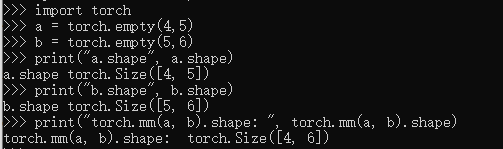2. 三维带batch的矩阵乘法 torch.bmm()
torch.bmm(bmat1, bmat2, out=None)
由于神经网络训练一般采用 mini-batch，经常输入的是三维带 batch 的矩阵。 提供 torch.bmm(bmat1, bmat2, out=None)，
其中 bmat1

∈

R

b

a

t

c

h

×

m

×

n

\in \mathbb{R}^{batch \times m \times n}

，bmat2

∈

R

b

a

t

c

h

×

n

×

d

\in \mathbb{R}^{batch \times n \times d}

，out

∈

R

b

a

t

c

h

×

m

×

d

\in \mathbb{R}^{batch \times m \times d}

。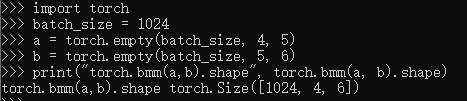3. 多维矩阵乘法 torch.matmul()
torch.matmul(input, other, out=None)
针对多维数据 matmul()乘法，我们可以认为该matmul()乘法使用使用两个参数的后两个维度来计算，其他的维度都可以认为是batch维度。
假设两个输入的维度分别是
input

∈

R

1000

×

500

×

99

×

11

\in \mathbb{R}^{1000 \times 500 \times 99 \times 11}

, other

∈

R

500

×

11

×

99

\in \mathbb{R}^{500 \times 11\times 99}

可以认为 torch.matmul(input, other, out=None) 乘法首先是进行后两位矩阵乘法得到(99×11)×(11×99)⇒(99×99)，然后分析两个参数的batch size分别是 (1000×500) 和 500 。
可以广播成为 (1000×500)， 因此最终输出的维度是 (1000×500×99×99)。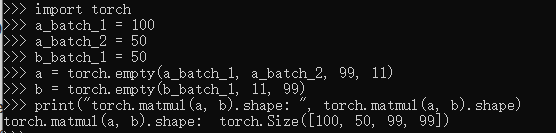4. 矩阵逐元素(Element-wise)乘法 torch.mul()
torch.mul(mat1, other, out=None)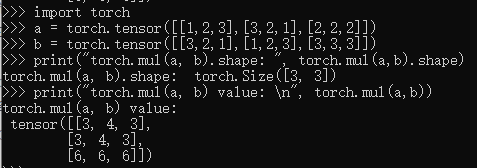5. 运算符重载
import torch
import numpy as np
a = torch.rand(2,3)
b = torch.rand(3)
# 这里b 使用了 broatcasting 自动进行了维度扩展
print("运算符 - 与 sub() 方法运算结果一致：{} ".format(torch.all(torch.eq(torch.sub(a,b),a-b))))
print("运算符 * 与 mul() 方法运算结果一致：{} ".format(torch.all(torch.eq(torch.mul(a,b),a*b))))
print("运算符 / 与 div() 方法运算结果一致：{} ".format(torch.all(torch.eq(torch.div(a,b),a/b))))


运算符 + 与 add() 方法运算结果一致：True
运算符 - 与 sub() 方法运算结果一致：True
运算符 * 与 mul() 方法运算结果一致：True
运算符 / 与 div() 方法运算结果一致：True


矩阵相乘 torch.matmul（只取最后两维度进行运算）， @(是matmul方法的重载) 两种方法
import torch
import numpy as np
a = torch.rand(2,3)
b = torch.rand(3,4)
print("运算符 @ 与 matmul 方法运算结果一致：{} ".format(torch.all(torch.eq(torch.matmul(a,b),a@b))))
print("运算后张量的 shape: {}".format((a@b).shape))


运算符 @ 与 matmul 方法运算结果一致：True
运算后张量的 shape: torch.Size([2, 4])


pow / ** 幂运算
import torch
import numpy as np
a = torch.full([2,2],2)

print("a 的二次方: {}".format(a.pow(2)))
print("a 的三次方: {}".format(a**3))
#平方根
print("a 的平方根: {}".format(torch.sqrt(a.pow(2))))

a 的二次方: tensor([[4., 4.],
[4., 4.]])
a 的三次方: tensor([[8., 8.],
[8., 8.]])
a 的平方根: tensor([[2., 2.],
[2., 2.]])


exp / log
import torch
import numpy as np
a = torch.exp(torch.ones(2,2))
print("e 为: {}".format(a))
print("e 取log : {}".format(torch.log(a)))

e 为: tensor([[2.7183, 2.7183],
[2.7183, 2.7183]])
e 取log : tensor([[1., 1.],
[1., 1.]])


clamp 范围限幅 （min）将低于min的值裁剪为min (min,max) 将数据低于min 裁剪为min,高于max裁剪为max
import torch
import numpy as np
a = torch.randn(2,3)
print("a 为: {}".format(a))
print("a 裁剪后为 : {}".format(a.clamp(0.1)))
print("a 裁剪后为 : {}".format(a.clamp(0.1,0.3)))


a 为: tensor([[-0.4780, -0.2077,  0.3702],
[-1.5801, -0.0170,  0.6737]])
a 裁剪后为 : tensor([[0.1000, 0.1000, 0.3702],
[0.1000, 0.1000, 0.6737]])
a 裁剪后为 : tensor([[0.1000, 0.1000, 0.3000],
[0.1000, 0.1000, 0.3000]])


展开全文深度学习 机器学习 神经网络 人工智能
• 在机器学习和深度学习中时，矩阵运算是最常见、有效提高计算效率的方法。因为特征和权重都以向量储存，矩阵运算就显得尤为重要，诸如梯度下降、反向传播、矩阵因子分解等重要的机器学习方法，都需要基于矩阵运算。 ...
在机器学习和深度学习中时，矩阵运算是最常见、有效提高计算效率的方法。因为特征和权重都以向量储存，矩阵运算就显得尤为重要，诸如梯度下降、反向传播、矩阵因子分解等重要的机器学习方法，都需要基于矩阵运算。
在深度学习中，神经网络将权重储存在矩阵当中，基于线性代数的运算在 GPU上，可以对矩阵进行简单迅捷的计算处理。

小规模的矩阵运算，可以用 for 循环来快速运算。但是一旦遇到巨大的数据量，循环语句就会拖慢运算速度，这时候我们常常使用矩阵乘法，它可以极大提高运算的效率。

常见矩阵、向量、标量相乘方法
torch(mm) 二维矩阵乘法
torch.mm(mat1,ma2,out=None), 其中 mat1(n*m), mat2(m*d), 输出 out 的维度是(n*d)。
注：计算两个二维矩阵的矩阵乘法，而且不支持广播。

torch.bmm() 三维带 Batch 矩阵乘法
torch.bmm(bmat1,bmat2,out=None)，其中 bmat1(b*n*m), bmat2(B*m*d), 输出 out 的维度是 (B*n*d)。
注：该函数的两个输入必须是三维矩阵且第一维相同（表示Batch维度），不支持广播。

torch.matmul() 混合矩阵乘法
torch.matmul(input,other,out=None)，该方法较为复杂建议参考文档，两个张量的矩阵乘积，计算结果取决于张量的维度，支持广播。

torch.mul() 矩阵逐元素乘法
torch.mul(mat1,other,out=None)，其中 other 乘数可以是标量也可以是任意维度的矩阵，只要满足最终相乘即可，支持广播。

torch.mv() 矩阵向量乘法
torch.mv(mat, vec, out=None) ，对矩阵 mat 和向量 vec 进行相乘。如果mat 是一个 n×m 张量，vec 是一个 m 元的一维张量，将会输出一个 n 元的一维张量，不支持广播。

torch.dot() 张量点乘法
torch.dot(tensor1, tensor2) ，计算两个张量的点乘,两个张量都为 1-D 向量，不支持广播。

论坛热门问题
Q：不用 for 循环，如何进行批数据向量相乘 ？
input shape：N x M x VectorSize
weight shape：M x VectorSize x VectorSize
目标 output shape：N x M x VectorSize
N 表示批尺寸，M 表示向量数量，VectorSize 则代表向量的大小。
如下图所示：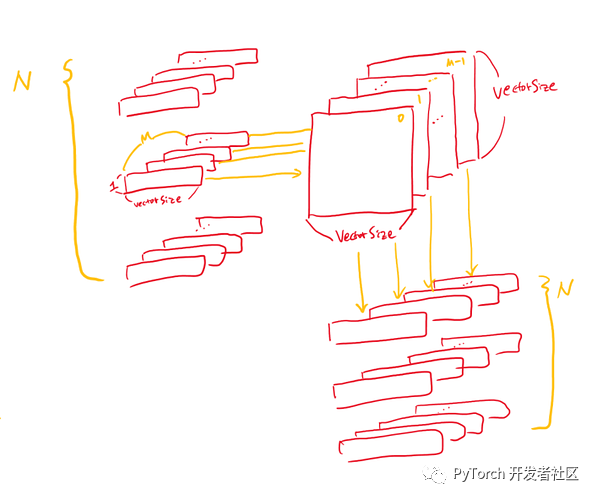解决思路：
N, M, V=2, 3, 5a = torch.randn(N, M, V)b = torch.randn(M, V, V)a_expand = a.unsqueeze(-2)b_expand = b.expand(N, -1, -1, -1)​c = torch.matmul(a_expand, b_expand).squeeze(-2)

原帖地址：
https://discuss.pytorch.org/t/batch-matrix-vector-multiplication-without-for-loop/112841

Q：计算矩阵向量乘以批向量输入，出现 RuntimeError 报错
给定:
# (batch x inp)v = torch.randn(5, 15)# (inp x output)M = torch.randn(15, 20)

计算:
# (batch x output)out = torch.Tensor(5, 20)for i, batch_v in enumerate(v):    out[i] = (batch_v * M).t()

但是 (i) 乘法对于两个维度相同的输入，都会出现：
RuntimeError: inconsistent tensor size at /home/enrique/code/vendor/pytorch/torch/lib/TH/generic/THTensorMath.c:623
为什么会出现这个报错？有没有能避免行向量循环的方法？

解决思路：
* 表示 elementwise 乘法，如果你用的是 Python3，可以使用 @ 算子可以用作矩阵-向量乘法和矩阵-矩阵乘法。
也可以使用 batch_v.mm(M)，因为批向量矩阵（batched vector matrix）即矩阵-矩阵乘法。
此外还可以借助 bmm、 baddbmm 等方法消除 Python 中的循环操作。

原贴地址：
https://discuss.pytorch.org/t/matrix-vector-multiply-handling-batched-data/203

Q：不使用循环语句的前提下，如何计算矩阵向量相乘？
求 2x2 的矩阵乘以 3 个大小为 2 的向量，如果不使用循环，该如何实现？
备注：
1、使用循环语句时代码如下:
A=torch.tensor([[1.,2.],[3.,4.]])b=torch.tensor([[3.,4.], [5.,6], [7.,8.]])res = []for i in range(b.shape):    res.append(torch.matmul(A, b[i]))

2、经尝试 torch.matmul (A.repeat ((2,1,1)) ，b) 行不通。
解决思路：
可以通过 a.matmul (b.t ()) 实现。
原帖地址：
https://discuss.pytorch.org/t/vectorize-matrix-vector-multiplication/88051
本公众号将持续更新 PyTorch 最新资讯和开发技巧，欢迎关注！展开全文深度学习 机器学习 人工智能
• 在查看fastnlp实现的CRF时，在前向传播过程中计算emit_score时遇到了加法广播操作，如下所示 tmp = alpha.view(batch_size, tag_num, 1) + emit_score + trans_score # 上一个位置得分加上当前得分 ''' 其中，alpha...
• 广播（broadcast）规则 对于两个数组，对于它们的维度元组倒序看，当两者在当前维度...高维度矩阵广播也可以按照这个来分析。 示例 Pytorch中的视图方法(view) def squared_loss(y_hat, y): return (y_hat - y.v...
• torch.mm(a, b) 是矩阵a和b矩阵相乘，比如a的维度是(1, 2)，b的维度是(2, 3)，返回的就是(1, 3)的矩阵。 torch.bmm() 强制规定维度和大小相同 torch.matmul() 没有强制规定维度和大小，可以用利用广python
• 1、矩阵相乘（1）二维矩阵乘法：torch.mm(mat1, mat2, out=None) → Tensor 该函数一般只用来计算两个二维矩阵矩阵乘法，并且不支持broadcast操作。 代码例子：对应行列乘 In: mat1 = t.Tensor([[1,2,3],[2,...点乘 mul
• ## Pytorch广播是什么？

千次阅读 2017-08-17 10:15:39
其实和Numpy的广播是差不多的，就是改变小矩阵的形状为大矩阵的形状然后运算。具体参考：使用Pytorch广播语义实现不同形状数组的运算 - pytorch中文网 原文出处： https://ptorch.com/news/39.html
• 1.pytorch广播机制与numpy类似，当两个tensor逐元素相乘时（两个...在pytorch中，*表示逐元素相乘，而@是矩阵乘积，但是对于高维tensor，@不再适用，尤其是对于size大小还不同的两个张量，pytorch还有一个函数：mu机器学习
• 那么为了避免这些不易察觉的错误，一定要了解下广播机制。 众所周知， == 维度相同，形状相同的数组可以进行数值运算。== 图中a是2行3列的二维数组，b是2行3列的数组。维度相同，都是二维数组。 形状相同，都是2行3...深度学习
• Pytorch 中 matmul 广播方式 一、两个 1 维，向量内积 a = torch.ones(3) b = torch.ones(3) print(torch.matmul(a,b)) # tensor(3.) 二、两个 2 维，矩阵相乘 a = torch.ones(3,4) b= torch.ones(4,3) ...
• ## pytorch中的广播机制

千次阅读 多人点赞 2020-01-02 17:07:04
pytorch中的广播机制和numpy中的广播机制一样, 因为都是数组的广播机制 两个维度不同的Tensor可以相乘, 示例 a = torch.arange(0,6).reshape((6,)) ''' tensor([0, 1, 2, 3, 4, 5]) shape: torch.Size() ndim: 1 ...python
• 1. 二维矩阵乘法 torch.mm() torch.mm(mat1, mat2, out=None) 其中mat1(nxm), mat2(mxd), 输出out(nxd) 一般只用来计算两个二维矩阵矩阵乘法，而且不支持broadcast操作。 torch.mm(input, mat2, out=None) → ...
• torch.mul和直接用运算符 * 都是点乘（可广播） torch.mm只能二维矩阵相乘，而torch.matmul可以广播到多维 参见： https://blog.csdn.net/weixin_42105432/article/details/100691592
• #官方提示：不能进行广播（broadcast）. #example >>> torch.dot(torch.tensor([2, 3]), torch.tensor([2, 1])) #即对应位置相乘再相加 tensor(7) >>> torch.dot(torch.rand(2, 3), torch.rand(2,...线性代数
• print("c1.shape: ", c1.shape) a2 = torch.rand(4, 1, 28, 64) b2 = torch.rand(4, 3, 64, 32) c2 = torch.matmul(a2, b2) # 这里用到了广播机制 print("c2.shape: ", c2.shape) 幂运算 import torch a = torch....深度学习
• 2）如果两个都是2维的，就矩阵相乘3）如果第一个是1维，第二个是2维；填充第一个使得能够和第二个参数相乘；如果第一个是2维，第二个是1维，就是矩阵和向量相乘；例：a = torch.zeros(7,)b= torch.zeros(7,8) >&...
• pytorch矩阵相加时，会自动根据矩阵的阶数进行自动扩展。 在一定条件下，可用让阶数不同的矩阵相加。 示例： import torch a1 = torch.tensor([ [0, 0, 0], [10, 10, 10], [20, 20, 20], [30, 30, 30] ]) b1...人工智能 python
• torch中的矩阵乘法: 一.矩阵乘 二.矩阵乘 三.对应元素相乘 torch.mul: 和普通标量也是对应元素相乘: #输入张量和标量相乘 torch.mul(input, value, out=None) 例： >>> a = torch...
• 导语：这是一篇关于Pytorch中各类乘法操作的总结和使用说明。torch.dot()：Computes the dot product (inner product) of two tensors.计算两个1-D 张量的点乘(内乘)。torch.dot(torch.tensor([2, 3]), torch.tensor...
• pytorch 与 numpy 的数组广播机制 </h1> <div class="clear"></div> <div class="postBody"> numpy 的文档提到数组广播机制为： When operating on two arrays, Num...
• 这是 Pytorch 里的一个知识点。由于Torch 的底层，参考了很多 numpy 的设计理念。所以也带入了很多 numpy 才有独有的概念。其中最主要的三个特性，广播机制、数据访问，以及按轴方向计算。由于 torch 中很多操作可能...索引...